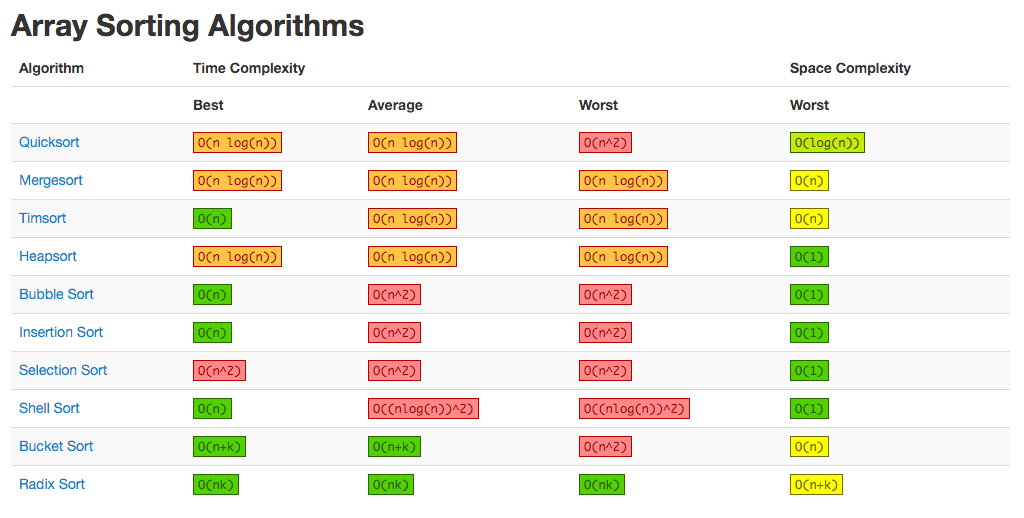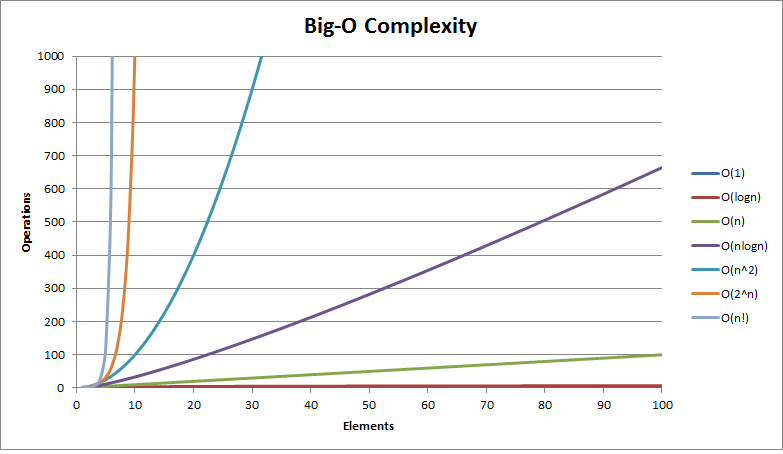Sorting algorithms in JavaScript

#sorting-algorithms series

Posted on March 12, 2016

The #sorting-algorithms series is a collection of posts about reimplemented sorting algorithms in JavaScript.

If you are not familiar with sorting algorithms, a quick introduction and the full list of reimplemented sorting algorithms can be found in the introduction post of the series on sorting algorithms in JavaScript.

If you feel comfortable with the concept of each sorting algorithm and only want to see the code, have a look at the summary post of the series. It removes all explanations and contains only the JavaScript code for all sorting algorithms discussed in the series.

Get the code on Github

Of course, all the code can also be found on Github in the repository sorting-algorithms-in-javascript.

A good way to compare all of them

Unlike the data structures, all sorting algorithms have the same goal and they can all take the same input data. So, for every sorting algorithms of the series, we are going sort an array of 10 numbers from 1 to 10.

By doing so we will be able to compare the different sorting algorithms more easily. Sorting algorithms are very sensitive to the input data so we will also try different input data to see how they affect the performances.

What is a sorting algorithm?

Instead of trying to re-explain what a sorting algorithm is, I prefer to leave you with the perfect description wikipedia makes of it.

A sorting algorithm is an algorithm that puts elements of a list in a certain order. Efficient sorting is important for optimizing the use of other algorithms (such as search and merge algorithms) which require input data to be in sorted lists; it is also often useful for producing human-readable output. From Wikipedia

Don’t stop to the obvious of the first sentence here. Indeed, it doesn’t seem so obvious for us human why sorting is so important because most of the time it is invisible for us. The definition mentions the use case for human readable output which is the only one that we are able to see but the use of optimizing other algorithms is for me even more important.

Sorting algorithms have been studied for decades, for example Bubble sort was analyzed as early as 1956, and are still today. They also are a very good introduction to computer science because they allow to introduce severals algorithm concepts with a very simple goal. This is another reason why it is good to study them.

Finally, as for the data structures, remember that each sorting algorithm has it own advantages and disadvantages. There isn’t any one of them that would beat all of the others, that’s the reason why it is important to know them all.

The complexity

If you hear about sorting algorithms, you will for sure hear about their complexity. As said before every sorting algorithms has its own advantages and disadvantages, their complexity can be seen as a way of expressing their own advantages and disadvantages easily according to a specific set of input data.

The complexity for sorting algorithms is probably even more important than for data structures. Data structures can be chosen according to some specific needs of your application but the use case for sorting algorithms is almost always the same. Therefore it is even more important to know which is the underlying complexity for such or such algorithm according to a set of input data.

The complexity is expressed on 2 axes, the space and the time.

The space

The space complexity represents the memory consumption of a sorting algorithm. As for most of the things in life, you can’t have it all, so it is with the sorting algorithms. You will generally need to trade some time for space or the other way around.

The time

The time complexity for a sorting algorithm is in general more diverse than its space complexity.

Only one type of operation

In contrary to data structures, when you look at the time complexity for sorting algorithms you only have one operation which is sorting. Therefore the focus, when speaking of time complexity for sorting algorithms, is often put on the different use cases (average, best and worst).

Dependent on data

Something that data structure and sorting algorithms have in common when talking about time complexity is that they are both dealing with data. When you deal with data you become dependent on them and as a result the time complexity is also dependent of the data that you received. To solve this problem we talk about 3 different time complexity.

• The best-case complexity: when the data looks the best
• The worst-case complexity: when the data looks the worst
• The average-case complexity: when the data looks average

As the operation for sorting algorithms is unique, you obtain theses different use cases by varying the set of input data. You can think for example to an already sorted array, or a reversed sorted array, etc…

The big O notation

The complexity is usually expressed with the Big O notation. The wikipedia page about this subject is pretty complex but you can find here a good summary of the different complexity for the most famous data structures and sorting algorithms.